Register for Maths, Science, English, Reasoning Olympiad Exams Register here | Book Free Demo for Live Olympiad Classes here | Check Olympiad Exam Dates here | Buy Practice Papers for IMO, IOM, HEO, IOEL etc here | Login here to participate in all India free mock test on every Saturday

# Fractions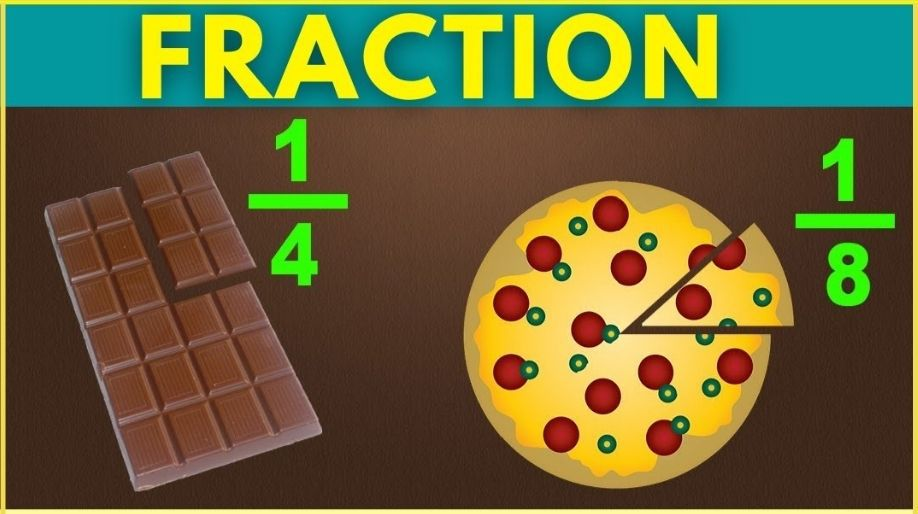• What is fraction? A fraction is a numerical quantity that is not a whole number.
For example,
½ is a fraction of
1 as numerator and
2 as a denominator

Fractions having the same denominator are called like fractions.
For example,
½,3/2, 5/2, 7/2, are
all like fractions.

• Fractions having different denominators are called, unlike fractions.
For example,
½, 2/3, ¾, 4/5,
are all unlike fractions
• A fraction whose numerator is less than the denominator is called proper fraction.
For example,
8/9, 7/8, 6/7, 5/6
are all proper fractions.
• A fraction whose numerator is greater than the denominator is called improper fraction.
For example,
3/2, 4/3, 5/4, 6/5
are all improper fractions.

Fractions which represent same or equal values are called equivalent
fractions.
For example,
1/3, 2/6, 3/9, 4/12
are all equivalent fractions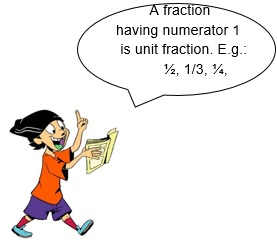SOLUTION: Total Part= 8 Fraction of shaded part= 3/8 Fraction of unshaded part= 5/8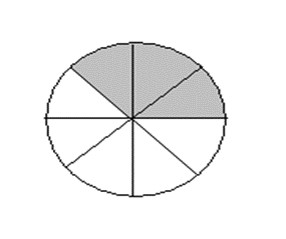EXAMPLE 2: Find the fraction of red balls, green balls and blue balls.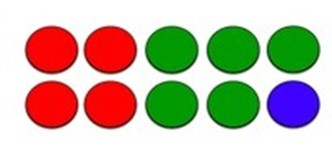SOLUTION: Total number of balls= 10 Number of red balls= 4

Fraction of red balls= 4/10= 2/5

Fraction of green balls= 5/10= ½

Fraction of blue balls= 1/10

Fraction as a division

• Any fraction can be expressed as a division by writing its numerator as dividend and denominator as divisor

Numerator/Denominator

= Dividend ÷ Divisor

=Dividend/Divisor

EXAMPLE 1: Write 1÷2 as a fraction.

SOLUTION: ½

EXAMPLE 2: Write 2/3 as division.

SOLUTION: 2÷3

To convert a mixed no. into an improper fraction & vice versa

• To convert a mixed number into an improper fraction multiply the quotient with the divisor and add the product with remainder in the numerator. The denominator will contain the divisor.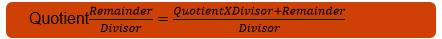• To convert an improper fraction into a mixed number, divide the numerator of the fraction by the denominator. Write the quotient as the whole number. The remainder in the numerator and the divisor in the denominator.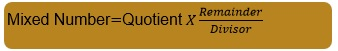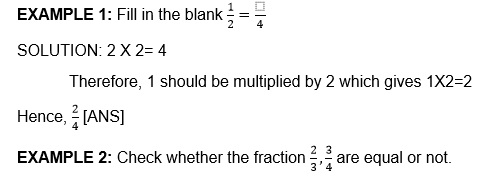Finding and checking equivalent fraction

• To find the equivalent fraction to a given fraction, divide or multiply the numerator or denominator by the same number. (other than zero)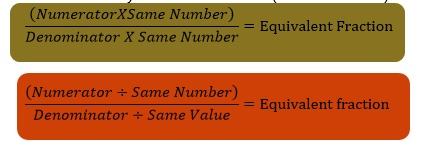• To check for equivalent fractions, we need two equivalent fraction.SOLUTION: 2x4=8 3X3=9 8≠9

Hence, the fractions are not equal.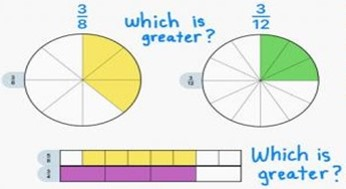Find the greater fraction!

To find a fraction in its lowest term

• A fraction is in its lowest term when the numerator and the denominator don’t have a common factor, except 1.
• There are two methods of finding a fraction in its lowest term. They are:
Method 1:
Divide the numerator
and denominator with their
common factor till we are left
with only the common factor 1

Method 2:
Divide the numerator
and denominator of the
given fraction with their HCF.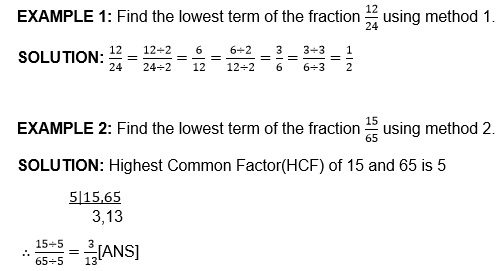To find the fraction of a number or quantity

• Divide the number by the denominator. Then, multiply the quotient so obtained by the numerator.

EXAMPLE 1: A group has 120 children. 4/5 of them are girls. Find the number of boys.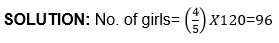No. of boys= (120-96) = 24

EXAMPLE 2: Find 1/4 of a year in months.

SOLUTION: A year has 12 months.
¼ X 12 = 3 months [ANS]

To compare unlike fractions

• First find the LCM of the denominators of the given fractions.
• Then convert the unlike fractions into equivalent like fraction with LCM as their common denominator.
• Compare the like fractions.

Convert mixed fractions
into improper fractions to
compare them.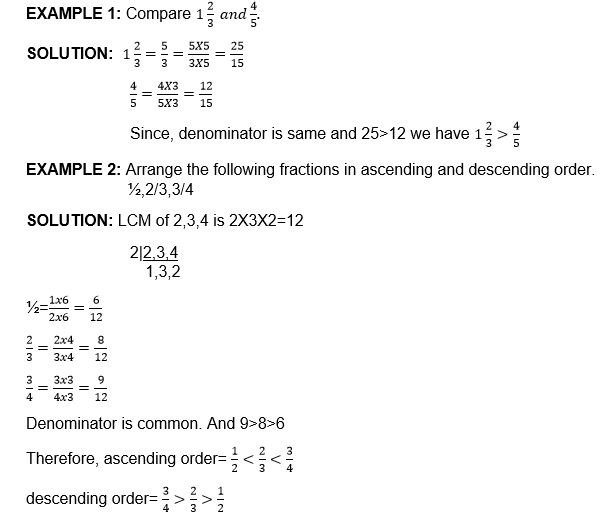• Find the LCM of the denominator of unlike fraction.
• Then convert the unlike fraction into equivalent like fraction with LCM as common denominator.
• Add/subtract the like fraction so obtained.

EXAMPLE 1: Add/subtract ½ and/from 1/6.

SOLUTION: LCM of 2 and 6 is = 2x3=6 2|2,6 1,3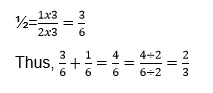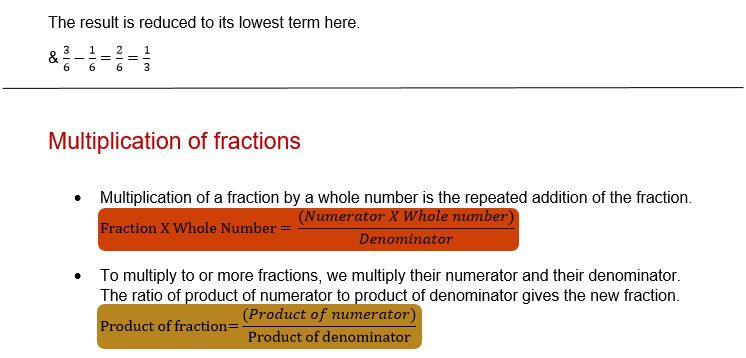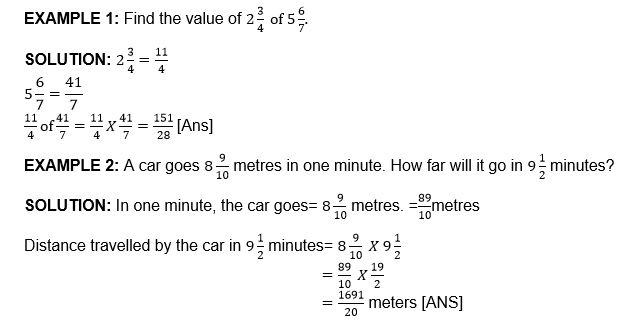# Reciprocal of a fractional number

• When the product of two fraction is 1, we say that each of the fraction is the reciprocal or multiplicative inverse of the other.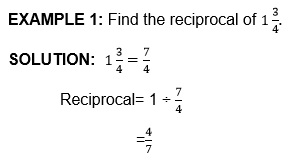Division of fractions

• Division is repeated subtraction.
• Division by a fraction is same as multiplication by its reciprocal.
0 has no reciprocal.
The reciprocal of 1 is 1.
0 divided by any non-zero number = 0Practice these questions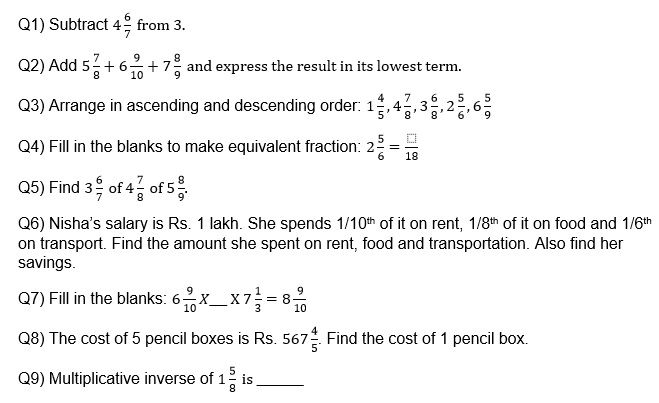Recap

• LCM of denominators is to be found only while performing addition or subtraction of unlike fractions.
• While multiplying fractions we can change their order, but the product remains the same. (commutative property)
• If a fraction is multiplied by 0, the product is always zero.
• If a fraction is multiplied by 1, the product is the same fraction.
• A fraction is in the lowest term when the only common factor between the numerator and the denominator is 1
• If any of the fraction is a mixed number or a whole number, change it to an improper function and then multiply.

## Quiz for Fractions

 Q.1 Which one is a proper fraction? a) 44/11 b) 24/6 c) 19/78 d) 7 1/11
 Q.2 Which one is a improper fraction? a) 45/17 b) 16/38 c) 14/128 d) 5 5/120
 Q.3 What is the mixed fraction of 23/3? a) 7 + 2/3 b) 2+7/3 c) 4/7+5 d) 5+2/7
 Q.4 Find the improper fraction of 7+(12/60) a) 12 b) 8 c) 34/5 d) 36/5
 Q.5 Fill in the blank. 7/6 = __ a) 14/6 b) 21/18 c) 21/12 d) 18/21
 Q.6 Rahul ate 3 pieces of Pizza. Raj ate 2 pieces of Pizza. If there are initially 10 pieces then what fraction of Pizza is left? a) (1/2) b) (1/5) c) (5/2) d) none of the above
 Q.7 Marry and Steve are brother & sister. They have a computer. Marry fills 3/5 of hard disk with her data and Steve fills 1/3 of hard disk with his data. What fraction of the hard disk is empty? a) 14/15 b) 1/15 c) 2/5 d) 2/3
 Q.8 Fill in the blank. 69/7 __ 77/7. a) >= b) = c) > d) <
 Q.9 Consider the values: 8/16, 7/14, 42/21, 96/3, 12/4. Find out which one is the highest value. a) 96/3 b) 12/4 c) 42/21 d) 7/14
 Q.10 Consider the values 77/7, 45/5, 125/25, 33/18. Find out which one is the lowest value. a) 125/25 b) 33/18 c) 45/5 d) none of the above